# Adding (blending) two images using OpenCV¶

## Goal¶

In this tutorial you will learn:

• what is linear blending and why it is useful;

## Theory¶

Note

The explanation below belongs to the book Computer Vision: Algorithms and Applications by Richard Szeliski

From our previous tutorial, we know already a bit of Pixel operators. An interesting dyadic (two-input) operator is the linear blend operator: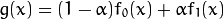By varying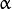from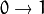this operator can be used to perform a temporal cross-dissolve between two images or videos, as seen in slide shows and film productions (cool, eh?)

## Code¶

As usual, after the not-so-lengthy explanation, let’s go to the code:

#include <cv.h>
#include <highgui.h>
#include <iostream>

using namespace cv;

int main( int argc, char** argv )
{
double alpha = 0.5; double beta; double input;

Mat src1, src2, dst;

/// Ask the user enter alpha
std::cout<<" Simple Linear Blender "<<std::endl;
std::cout<<"-----------------------"<<std::endl;
std::cout<<"* Enter alpha [0-1]: ";
std::cin>>input;

/// We use the alpha provided by the user if it is between 0 and 1
if( input >= 0.0 && input <= 1.0 )
{ alpha = input; }

/// Read image ( same size, same type )

/// Create Windows
namedWindow("Linear Blend", 1);

beta = ( 1.0 - alpha );
addWeighted( src1, alpha, src2, beta, 0.0, dst);

imshow( "Linear Blend", dst );

waitKey(0);
return 0;
}


## Explanation¶

1. Since we are going to perform:We need two source images (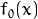and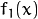). So, we load them in the usual way:

src1 = imread("../../images/LinuxLogo.jpg");


Warning

Since we are adding src1 and src2, they both have to be of the same size (width and height) and type.

2. Now we need to generate the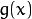image. For this, the function addWeighted comes quite handy:

beta = ( 1.0 - alpha );
addWeighted( src1, alpha, src2, beta, 0.0, dst);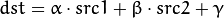In this case,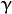is the argument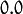in the code above.

3. Create windows, show the images and wait for the user to end the program.

## Result¶